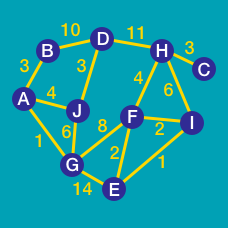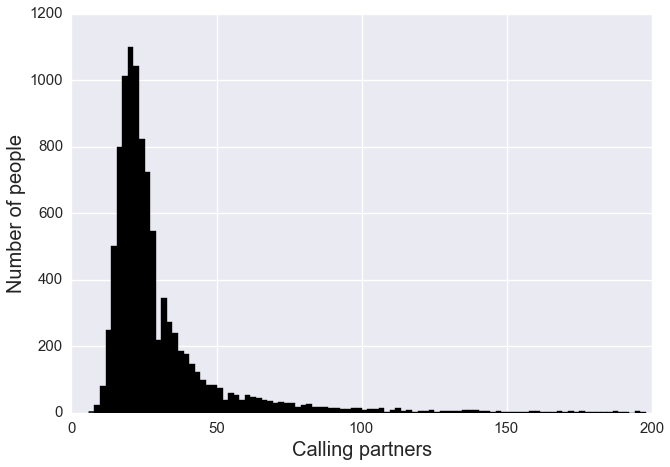Computer Science

# Depth-first SearchNSA collects metadata for a large fraction of the cell phone calls placed in the United States. Upon inspection, analysts are allowed to make three hops from a targeted calling account. In other words, they're allowed to inspect the metadata for every contact that the targeted person has made, for every contact that those contacts have made, for every contact that those contacts have made, and finally, for all the contacts that those contacts have called, i.e. three "hops". One person in a community of 10,000 is targeted for having called a grandparent in Yemen.In addition, there are two toll free hotlines, one for emergency assistance that is called by 214 unique community members, and another for information about various community events that is called by 673 unique community members. The full calling record for the community is listed here.

You are a journalist who has been given the calling record by a whistleblower inside NSA. You know that the targeting has been carried out but you don't know the phone number of the targeted individual. To get a sense of how many people fall under the dragnet when this one person is targeted, find the number of 2-hop connections for the following phone numbers, and report the average: 1 , 17, 793, 1200, 3402

Assumptions

• Each line representing a call between two numbers. The two integers are the "phone numbers" of the two individuals involved in the call.
 1 2 3 4 5 6 7 8 caller1 caller 2 1 2312 1 555 1 2794 1 3057 1 4032 1 4609 1 5707 

• The number of unique contacts for each caller has been generated according to the best fit to a real distribution published by Sprint corporation.Consider the above diagram, perform a depth first search starting at $A$. Let $T_{n}$ be the number of tree edges, $B_{n}$ the number of back edges, and $F_{n}$ be the number of forward edges. What is the value of $(T_{n}+B_{n})-F_{n}$?

To guarantee the same solution, whenever deciding which node to pick, choose a node whose label occurs earliest in the alphabet.Curious George plays around on a planar grid. George can move one space at a time: left, right, up or down.

That is, from $(x, y)$ George can go to $(x+1, y)$, $(x-1, y)$,$(x, y+1)$, or $(x, y-1)$.

George can access any point $(x,y)$ where the sum of the digits of $|x|$ $+$ the sum of the digits of $|y|$ is $\leq 19$.

How many points can George access if he starts at $(0,0)$ including $(0,0)$ itself?

Explicit Examples

• $(59, 79)$ is inaccessible because $5 + 9 + 7 + 9 = 30$, and $30 > 19$
• $(-5, -7)$ is accessible because $|-5| + |-7| = 12 \leq 19$
• $(190,90)$ is not reachable, considering its neighbors are all not reachable.
×